On-line GuidesAll GuideseBook StoreiOS / AndroidLinux for BeginnersOffice ProductivityLinux InstallationLinux SecurityLinux UtilitiesLinux VirtualizationLinux KernelSystem/Network AdminProgrammingScripting LanguagesDevelopment ToolsWeb DevelopmentGUI Toolkits/DesktopDatabasesMail SystemsopenSolarisEclipse DocumentationTechotopia.comVirtuatopia.comAnswertopia.comHow To GuidesVirtualizationGeneral System AdminLinux SecurityLinux FilesystemsWeb ServersGraphics & DesktopPC HardwareWindowsProblem SolutionsPrivacy Policy## Expression Exercises

There are two sets of exercises. The first section, the section called “Basic Output and Functions”, covers simpler exercises to reinforce Python basics. The secoind section, the section called “Numeric Types and Expressions”, covers more complex numeric expressions.

### Basic Output and Functions

1. Print Expression Results. In Simple Expressions, we entered some simple expressions into the Python interpreter. Change these simple expressions into print statements.

Be sure to print a label or identifier with each answer. Here's a sample.

```print "9-1's * 9-1's = ", 111111111*111111111
```
2. Evaluate and Print Expressions. Write short scripts to print the results of the following expressions. In most places, changing integers to floating point produces a notably different result. For example `(296/167)**2` and `(296.0/167.0)**2`. Use long as well as complex types to see the differences.

• `355/113 * ( 1 - 0.0003/3522 )`

• `22/17 + 37/47 + 88/83`

• `(553/312)**2`

3. Numeric Conversion. Write a print statement to print the mixed fraction 3 5/8 as a floating point number and as an integer.

4. Numeric Truncation. Write a print statement to compute `(22.0/7.0)-int(22.0/7.0)`. What is this value? Compare it with `22.0/7.0`. What general principal does this illustrate?

5. Illegal Conversions. Try illegal conversions like `int('A')` or `int( 3+4j )`. Why are exceptions raised? Why can't a simple default value like zero or `None` be used instead?

6. Evaluate and Print Built-in Math Functions. Write short scripts to print the results of the following expressions.

• `pow( 2143/22, 0.25 )`

• `pow(553/312,2)`

• `pow( long(3), 64 )`

• `long( pow(float(3), 64) )`

Why do the last two produce different results? What does the difference between the two results tell us about the number of digits of precision in floating-point numbers?

7. Evaluate and Print Built-in Conversion Functions. Here are some more expressions for which you can print the results.

• hex( 1234 )

• int( hex(1234), 16 )

• long( '0xab' )

• int( '0xab' )

• int( '0xab', 16 )

• int( 'ab', 16 )

• cmp( 2, 3 )

### Numeric Types and Expressions

1. Stock Value. Compute value from number of shares × purchase price for a stock.

Once upon a time, stock prices were quoted in fractions of a dollar, instead of dollars and cents. Create a simple print statement for 125 shares purchased at 3 and 3/8. Create a second simple print statement for 150 shares purchased at 2 1/4 plus an additional 75 shares purchased at 1 7/8.

Don't manually convert 1/4 to 0.25. Use a complete expression of the form 2+1/4.0, just to get more practice writing expressions.

2. Convert Between °C and °F. Convert temperatures from one system to another.

Conversion Constants:  32°F = 0°C, 212°F = 100°C.

The following two formulae converts between °C (Celsius) and °F (Fahrenheit).

Equation 4.2. Convert °C (Celsius) to °F (Fahrenheit)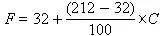Equation 4.3. Convert °F (Fahrenheit) to °C (Celsius)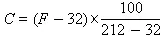Create a print statement to convert 18° C to °F.

Create a print statement to convert -4° F to °C.

3. Periodic Payment on a Loan. How much does a loan really cost?

Here are three versions of the standard mortgage payment calculation, with m =payment, p =principal due, r =interest rate, n =number of payments.

Equation 4.4. Mortage Payment, version 1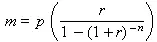Equation 4.5. Mortgage, payments due at the end of each period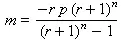Equation 4.6. Mortgage, payments due at the beginning of each period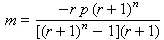Use any of these forms to compute the mortgage payment, m , due with a principal, p , of \$110,000, an interest rate, r , of 7.25% annually, and payments, n , of 30 years. Note that banks actually process things monthly. So you'll have to divide the interest rate by 12 and multiply the number of payments by 12.

4. Surface Air Consumption Rate. SACR is used by SCUBA divers to predict air used at a particular depth.

For each dive, we convert our air consumption at that dive's depth to a normalized air consumption at the surface. Given depth (in feet), d , starting tank pressure (psi), s , final tank pressure (psi), f , and time (in minutes) of t , the SACR, c , is given by the following formula.

Equation 4.7. Surface Air Consumption Rate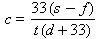Typical values for pressure are a starting pressure of 3000, final pressure of 500.

A medium dive might have a depth of 60 feet, time of 60 minutes.

A deeper dive might be to 100 feet for 15 minutes.

A shallower dive might be 30 feet for 60 minutes, but the ending pressure might be 1500. A typical c (consumption) value might be 12 to 18 for most people.

Write print statements for each of the three dive profiles given above: medium, deep and shallow.

Given the SACR, c , and a tank starting pressure, s , and final pressure, f , we can plan a dive to depth (in feet), d , for time (in minutes), t , using the following formula. Usually the 33( s - f )/ c is a constant, based on your SACR and tanks.

Equation 4.8. Time and Depth from SACRFor example, tanks you own might have a starting pressure of 2500 and and ending pressure of 500, you might have a c (SACR) of 15.2. You can then find possible combinations of time and depth which you can comfortably dive.

Write two print statements that shows how long one can dive at 60 feet and 70 feet.

5. Force on a Sail. How much force is on a sail?

A sail moves a boat by transferring force to its mountings. The sail in the front (the jib) of a typical fore-and-aft rigged sailboat hangs from a stay. The sail in the back (the main) hangs from the mast. The forces on the stay (or mast) and sheets move the boat. The sheets are attached to the clew of the sail.

The force on a sail, f , is based on sail area, a (in square feet) and wind speed, w (in miles per hour).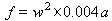For a small racing dinghy, the smaller sail in the front might have 61 square feet of surface. The larger, mail sail, might have 114 square feet.

Write a print statement to figure the force generated by a 61 square foot sail in 15 miles an hour of wind.

6. Craps Odds. What are the odds of winning on the first throw of the dice?

There are 36 possible rolls on 2 dice that add up to values from 2 to 12. There is just 1 way to roll a 2, 6 ways to roll a 7, and 1 way to roll a 12. We'll take this as given until a later exercise where we have enough Python to generate this information.

Without spending a lot of time on probability theory, there are two basic rules we'll use time and again. If any one of multiple alternate conditions needs to be true, usually expressed as “or”, we add the probabilities. When there are several conditions that must all be true, usually expressed as “and”, we multiply the probabilities.

Rolling a 3, for instance, is rolling a 1-2 or rolling a 2-1. We add the probabilities: 1/36+1/36 = 2/36 = 1/18.

On a come out roll, we win immediately if 7 or 11 is rolled. There are two ways to roll 11 (2/36) or 6 ways to roll 7 (6/36).

Write a print statement to print the odds of winning on the come out roll. This means rolling 7 or rolling 11. Express this as a fraction, not as a decimal number; that means adding up the numerator of each number and leaving the denominator as 36.

7. Roulette Odds. How close are payouts and the odds?

An American (double zero) roulette wheel has numbers 1-36, 0 and 00. 18 of the 36 numbers are red, 18 are black and the zeroes are green. The odds of spinning red, then are 18/38. The odds of zero or double zero are 2/36.

Red pays 2 to 1, the real odds are 38/18.

Write a print statement that shows the difference between the pay out and the real odds.

You can place a bet on 0, 00, 1, 2 and 3. This bet pays 6 to 1. The real odds are 5/36.

Write a print statement that shows the difference between the pay out and the real odds.Published under the terms of the Open Publication License Design by Interspire